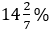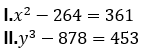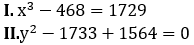SBI Clerk Quantitative Aptitude Quiz

With the increasing level in exams, quantitative aptitude has become the ineluctable hitch. Generally, questions asked in this section are calculative and lengthy that consumes your time. This subject can do wonders if you always keep a check on your accuracy, speed and time. Accuracy is what matters the most. To help you prepare the section we have provided a well-defined SBI Clerk Main 2019 study plan. You can also Prepare from the study notes to clear your basic concepts. Attempt this quantitative aptitude quiz and check your performance for the upcoming SBI Clerk exam 2019. Following is the quiz of 4th August, that inculcate the important topics from Line Di, Miscellaneous And Quadratic Equations.

Directions (1-5): Bar graph given below shows total employees (Officer + Clerk) working in five different banks and number of Clerk working in these banks respectively. Study the data carefully and answer the following questions.Q1. Find the ratio between Officers working in SBI and BOB together to Clerk working in UBI and OBC together?
None of the given options
21 : 31
17 : 31
17 : 29
21 : 29

Q2.Officers working in UBI and PNB together is what percent less than total employees (Officer + Clerk) working in OBC?
20%
80%
40%
None of the given options
60%

Q3.Find the average number of Officers working in all five banks together?
458
664
448
438
None of the given options

Q4. Male officers working in OBC is 40% more than female officers working in OBC while male clerk working in OBC is 50% less than female clerk working in OBC. Find total female employees (Officers + Clerks) working in OBC?
550
600
650
700
750

Q5. Find number of officers working in Allahabad bank if average number of officers working in SBI, BOB and Allahabad bank is 360?
380
400
None of the given options
390
420

Q6. A shopkeeper allows two successive discounts of 20% and 15% respectively on marked price of an article and customer also managed to get additional discount of Rs 220. Shopkeeper finds that selling price of the article is 53⅓% of marked price. Find cost price for the shopkeeper if he incurs a profit ofon it?
Rs. 735
Rs. 714
Rs. 665
Rs. 630
None of the above

Q7. Several litres of acid were drawn off a 54-litre vessel full of acid and an equal amount of water added. Again the same volume of the mixture was drawn off and replaced by water. As a result, the vessel contained 24 litres of pure acid. How much of the acid was drawn off initially ?
12 litres
16 litres
18 litres
24 litres
None of these

Q8. A container contains 80 lit of pure milk and X lit of water. Ratio of 25% of mixture to the initial quantity of water is 3 : 4 and when Z lit of water is added to it ratio of total mixture to water becomes 9 : 4 then find value of X+Z?
48 lit
72 lit
80 lit
64 lit
56 lit

Q9. A person buys some articles. He sold 40% of articles at 20% profit and remaining at 33⅓% profit. If percent profit is calculated on selling price then what is the ratio of selling price of articles sold at 20% profit to the articles sold at 33⅓% profit.
4 : 5
7 : 9
5 : 1
2 : 3
5 : 9

Q10. 18 litres of pure water was added to a vessel containing 80 litres of pure milk. 49 litres of the resultant mixture was then sold and some more quantity of pure milk and pure water was added to the vessel in the ratio of 2 : 1. If the resultant ratio of milk to water in the vessel was 4 : 1, what was the quantity of pure milk added to the vessel.
4
8
10
12
2

Directions (11-15): In each of these questions, two equations I and II are given. You have to solve both the equations and give answer

Q11.if x > y
if x ≥ y
if x < y
if x ≤ y
if x = y or no relation can be established between x and y

Q12.if x > y
if x ≥ y
if x < y
if x ≤ y
if x = y or no relation can be established between x and y

Q13.if x > y
if x ≥ y
if x < y
if x ≤ y
if x = y or no relation can be established between x and y

Q14.if x > y
if x ≥ y
if x < y
if x ≤ y
if x = y or no relation can be established between x and y

Q15.if x > y
if x ≥ y
if x < y
if x ≤ y
if x = y or no relation can be established between x and y